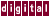# A.3 Input and Output Sets

To determine data dependence, it is necessary to form the input and output sets for the given statements.

`IN(S1)` denotes the set of input items of `S1` (items whose values may be read by `S1` ). `OUT(S1)` denotes the set of output items (scalar variables or array elements) of statement `S1` (items whose values may be changed by `S1` ).

The following example shows the `IN` and `OUT` sets for the assignment statement in the loop:

``` for (i=1; i<=10; i++)
S1:  x[i] = a[i+1] * b;

IN[S1] = {a, a, a, ..., a, b}

OUT[S1] = {x, x, x, ..., x}
```

In practice, KAP often approximates the `IN` and `OUT` sets because the actual loop bounds are frequently unknown at compile time.

Previous Page Next Page Contents Index
Command-Line Qualifiers